# Rooting Around in MATLAB – Part 2

In a recent post, I started a series of posts about finding roots of equations, and how you might use MATLAB to teach some of this topic. In particular, I will introduce the idea of fixed point iteration.

### Set Up

clear all
close all

### Example

Let me restate the example function. We'll start with a simple cubic polynomial f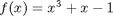which I define as an anonymous function.

f = @(x) x.^3 + x - 1;
fplot(f,[-2 2])
title f
grid on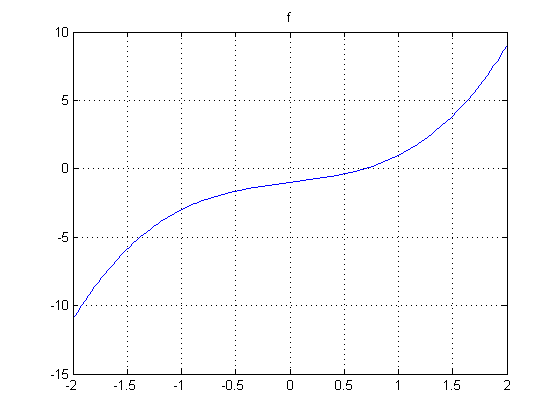### Fixed Point Algorithm

One way to find a solution to f(x) = 0 is to define another function, g such that g(x) = x where the function g is related to f. If we can find a value x that solves the equation with g, this fixed point is also the zero of the function f. How do we define such a suitable g? Looking at the equation for f and setting its value to zero, we can derive an equation for x.

Here's one way we can rewrite f(x) = 0 so that x is alone on one side of the equation.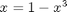Let's call this function g1.

g1 = @(x) 1 - x.^3;
fplot(g1,[-2 2]);
g1str = '1-x^3';
title g1
grid on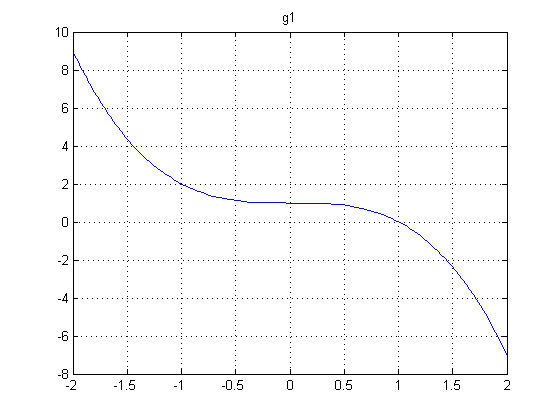Now let's add a straight line to represent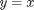straightLine = @(x) x;
title ''
hold on
fplot(straightLine, [-2 2], 'g')
legend(g1str,'x','Location','SouthEast')

axis([-2 2 -2 2]), axis equal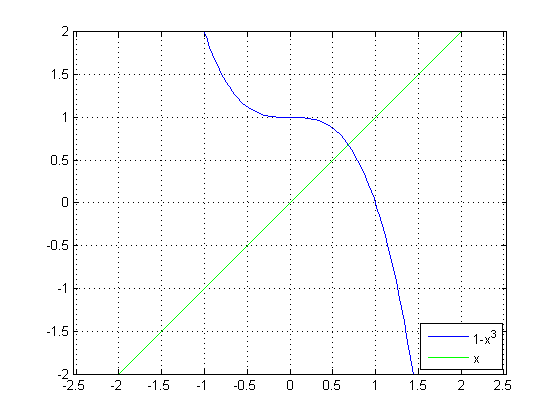The intersection of these 2 curves is a fixed point of g1(x), and therefore a zero of f(x). Let's zoom in a bit.

axis([0 1 0 1])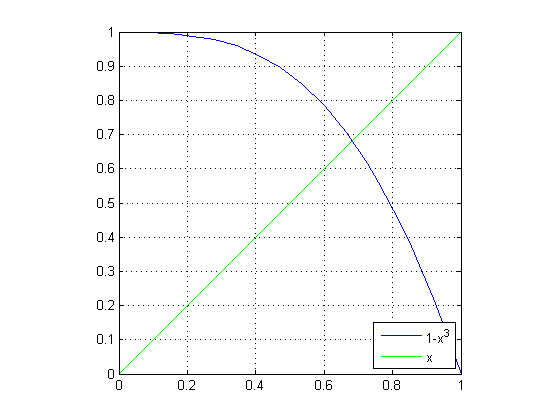That intersection looks like it happens around the same location as the answers we got from using roots and fzero in the previous post.

fzsolution = fzero(f,0.5)
fzsolution =
0.68233

plot(fzsolution, g1(fzsolution),'b*')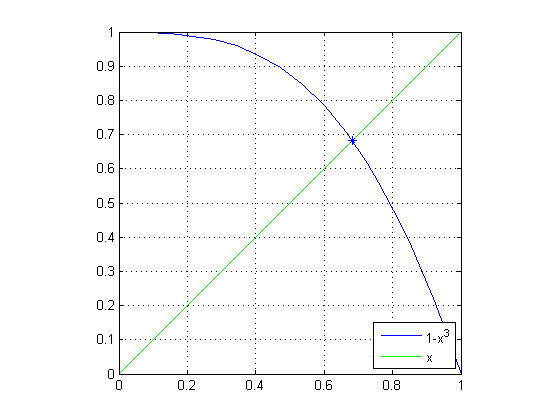### Let's Try to "Zero" in on the Solution

First, we'll select the same starting value that we used when we called fzero. The starting point is then (0.5,0.5). And then we can easily calculate the next value of g1.

x(1) = 0.5;
y(1) = x(1);
x(2) = x(1);
y(2) = g1(x(2));

Let's plot the trajectory of the solutions, noting the first point with a red star.

plot(x,y,'r',x(1),y(1),'r*')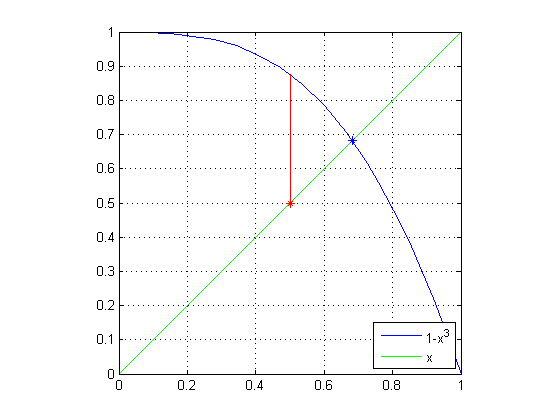### First Iteration

Now let's get the next estimate. We'll set the current value of g1 to the new x value, sliding onto the straight line, and then calculate the next value of g1 from this new value for x. And plot it.

x(3) = y(2);
y(3) = y(2);
x(4) = x(3);
y(4) = g1(x(4));
plot(x,y,'r')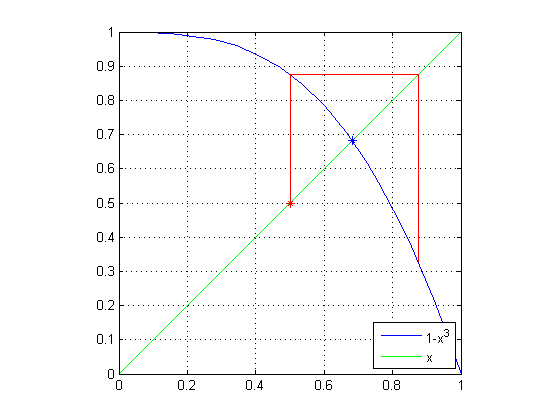### Second Iteration

Let's do the same set of steps again.

n = 5;
x(n) = y(n-1);
y(n) = y(n-1);
x(n+1) = x(n);
y(n+1) = g1(x(n+1));
plot(x,y,'r')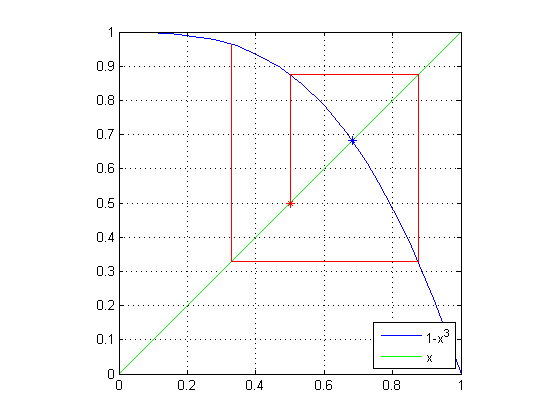### Third Iteration

And again.

n = 7;
x(n) = y(n-1);
y(n) = y(n-1);
x(n+1) = x(n);
y(n+1) = g1(x(n+1));
plot(x,y,'r')
hold off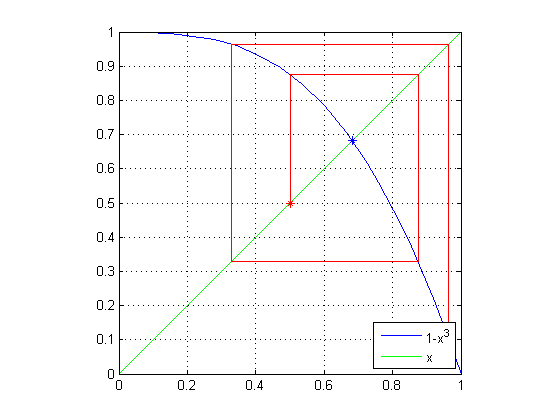### The Conundrum

By now, you have surely noticed that instead of spiraling into the solution we found with fzero, our estimates are spiraling out! In the next post, we'll explore another definition for g(x) = x and see what happens from there.

### Confession

Yes, I know in this post I kept overplotting lines successively. But I didn't want us to get distracted by managing the plots.

### The Series of Posts

In addition to this post and the previous one, there will be one more. Until it is published, the 3rd link will not be useful.

Published with MATLAB® 7.8

|